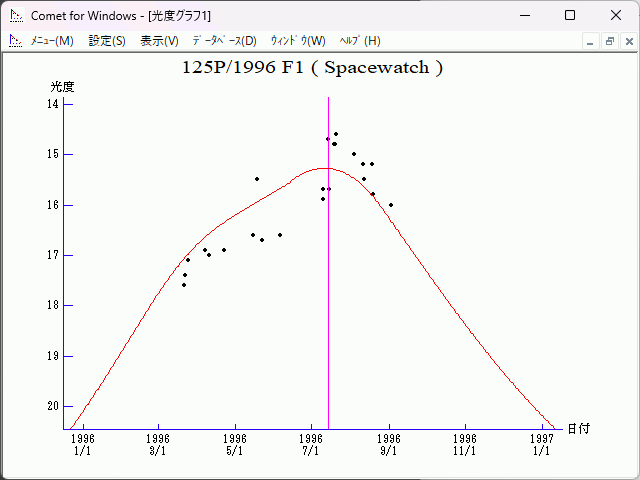# \$B%9%Z!<%9%&%)%C%AWB@1(B

125P/Spacewatch (1996)###\$B%W%m%U%#!<%k(B

 \$BId9f(B 125P/1996 F1 \$B8!=PF|(B 1996\$BG/(B3\$B7n(B21\$BF|(B \$B8!=P8wEY(B 17.6\$BEy(B \$B8!=P J. V. Scotti (Kitt Peak), J. Montani (Kitt Peak)

###\$B50F;MWAG(B

```   The following improved orbital elements by Kenji Muraoka, are
from 158 observations 1991 Sept. 8 to 1996 Sept. 2, perturbations
by 9 Planets, Moon and 5 minor planets were taken into account.
The mean residual is +/- 0.63 arc seconds.

Epoch  =  1996 July 16.0  TT       JDT = 2450280.5
T  =  1996 July 14.58862       +/- 0.00112 (m.e.) TT
Peri. =   87.27094                +/- 0.00019
Node  =  153.36897                +/- 0.00009   (2000.0)
Incl. =    9.96899                +/- 0.00002
q  =    1.5399039              +/- 0.0000015 AU
e  =    0.5090900              +/- 0.0000003
a  =    3.1368353              +/- 0.0000012 AU
n  =    0.17740527             +/- 0.00000010
P  =    5.556                  +/- 0.0000031  years
```

###\$B@1?^(B1996\$BG/(B 2\$B7n(B27\$BF|!A(B1996\$BG/(B12\$B7n(B23\$BF|(B

###\$B8wEYJQ2=(B

```        m1 = 14.0 + 5 log\$B&\$(B + 13.0 log r          [   ,-55]  (              \$B!A(B1996\$BG/(B 5\$B7n(B20\$BF|(B)
m1 =  6.4 + 5 log\$B&\$(B + 45   log r(t - 20)  [-55,112]  (1996\$BG/(B 5\$B7n(B20\$BF|!A(B1996\$BG/(B11\$B7n(B 3\$BF|(B)
m1 = 14.0 + 5 log\$B&\$(B + 13.0 log r          [112,   ]  (1996\$BG/(B11\$B7n(B 3\$BF|!A(B              )
```##### \$B50F;MWAG\$OB<2,7r<#;a\$N7W;;\$K\$h\$k\$b\$N\$G\$9!#(B \$B@1?^\$O(B StellaNavigator Ver.2.0 for Windows (\$B%"%9%H%m%"!<%D(B \$BJTCx(B / \$B%"%9%-!<=PHG6I4)(B) \$B\$G:n@.\$7\$?\$b\$N\$G\$9!#(B \$B8wEY%0%i%U\$O(BComet for Windows\$B\$G:n@.\$7\$?\$b\$N\$G\$9!#(B